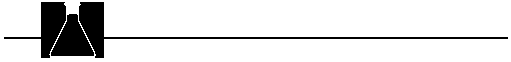## Demonstrations

Demonstration 1: A Molar Display

Purpose

To illustrate that one-mole samples of different substances have different volumes and masses.

Materials

One mole of various substances such as:
• Water, H2O, 18.0 g (18.0 mL)
• Methanol, CH3OH, 32.0 g (40.4 mL)
• Copper (use copper dust), Cu, 63.6 g
• Sodium chloride (table salt), NaCl, 58.5 g
• Carbon (activated charcoal), C, 12.0 g
• Sulfur, S, 32.1 g
• Iron (iron filings) Fe, 55.8 g Sucrose (table sugar), C12H22O11 , 342 g

Safety

No special precautions are needed if the above-listed substances are used.

Procedure

Display one mole of several different substances in identical containers, pointing out that one-mole samples of different substances have different volumes. Weigh one mole of several different substances to illustrate that moles of different substances have different masses. Suggestions of substances to use: water, methanol (or other alcohol), sucrose, salt, carbon, sulfur, iron filings, copper dust or other solids that are finely divided so the observed volume approximates the volume of the solid.

Demonstration 2: Molar Measurements

Purpose

To illustrate that the mole is a unit of measurement. (Extension of above demonstration.) This would also be an appropriate follow-up demonstration to the Understanding the Mole activity.

Materials

One mole and multiples and submultiples of a mole of a particular substance. For sodium chloride (table salt), NaCl, possible quantities are:

• NaCl (0.100 mol), 5.85 g
• NaCl (0.500 mol), 29.2 g
• NaCl (1.00 mol), 58.5 g
• NaCl (2.00 mol), 117 g
• NaCl (5.00 mol), 292 g
• NaCl (10.0 mol), 585 g

Safety

No special precautions are required if NaCl is used.

Procedure

Weigh one mole of a substance-for example sodium chloride or granular metal (Fe, Zn, etc.)-and arrange in a pile or heap. Label the pile "one mole NaCl" (or "one mole Zn," etc.) Prepare several other piles of the same substance to represent different numbers of moles (1/2, 2, 3, 5, etc.); ask students to guess the number of moles of substance in each pile. Use this demonstration to discuss the concept that the mole is a way to measure substances-if one knows the amount that one mole represents, one can determine how many moles are present in quantities larger or smaller than one mole. (You could use sugar or salt packets and have students empty the contents and guess the number of moles. They could then weigh the substance and calculate the number of moles to check their answer. If desired, students could also calculate molecules of sugar, grams of carbon present, etc. )

Since "mole" means "pile" or "heap" and molecule means "smallest part of the pile or heap," you could also use this demonstration to highlight the difference between the terms "mole" and "molecule." Of course, students cannot see a molecule (or atom) of the substance, but if you use a granular substance, they can see that there are many "grains" in a pile and must therefore be many more molecules in a pile.

Demonstration 3: How High the Mole

Purpose

To help students visualize the size of Avogadro's number.
Materials

Mole Demo, a Seraphim computer program (see reference to AP 305, AR 301, PC 2301 in Media section)
Safety
No safety precautions are necessary
Procedure
The Mole Demo computer program illustrates the stacking of sheets of paper from earth to Pluto. Use this after introducing the mole and Avogadro's number to help students realize the magnitude of Avogadro's number.
Remarks
This program requires about 5 min. Since there is no dialog (but musical sound effects occur periodically), consider preparing your own running commentary to accompany the program.

Demonstration 4: Moles and Gases

This demonstration is on the Ring of Truth: Atoms videotape and is also illustrated on CHEM Study video Gases and How They Combine (see references in Media section).

Purpose

To illustrate the relation between gas volume and moles of gas.

Materials

• Electrolysis apparatus (an inexpensive electrolysis apparatus is available from scientific supply vendors)
• Distilled water
• 0.1 M Sulfuric acid, H2SO4, several milliliters (to serve as electrolyte to facilitate electrolysis)
• Wooden splint (and match to ignite splint)

Safety

Observe usual safety precautions in handling the sulfuric acid. Follow directions supplied with electrolysis apparatus to set up the demonstration. Although the danger is slight for the small quantity of hydrogen produced in the electrolysis demonstration, the hydrogen could explode if ignited.

Procedure

An understanding of stoichiometry (or at least the meaning of formulas) is needed for students to understand this demonstration. Since the electrolysis of water produces two moles of hydrogen gas for every mole of oxygen gas, the volume of hydrogen formed in the electrolysis is twice the volume of oxygen. Consult directions accompanying the electrolysis apparatus to conduct the electrolysis demonstration. Collect 25-50 mL of the gases during electrolysis, or enough for the class to see that the volume of one gas is approximately twice the volume of the other. The gases collected can be tested to verify which is oxygen.

Ask the class to decide which gas is oxygen on the basis of the stoichiometry for the reaction (or the formula for water). Test only that gas. (Insert a glowing splint into a sample of the gas. If the splint bursts into flame, the gas is oxygen.) Warning: Do not attempt to ignite hydrogen as a proof that the other gas is hydrogen. Students will accept that the other electrolysis product must be hydrogen if water is electrolyzed.